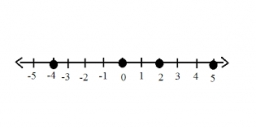# Divisors 7779

Two numbers are guessing. The second number is five times greater than the first number, and the square of the first number is equal to 3/5 of the second number, find the sum of the two numbers and all its divisors.

s1 =  18
d1 = 1, 2, 3, 6, 9, 18
s2 =  0
d2 =  0

### Step-by-step explanation:Did you find an error or inaccuracy? Feel free to write us. Thank you!

Tips for related online calculators
Are you looking for help with calculating roots of a quadratic equation?
Need help calculating sum, simplifying, or multiplying fractions? Try our fraction calculator.
Check out our ratio calculator.
Do you have a linear equation or system of equations and looking for its solution? Or do you have a quadratic equation?